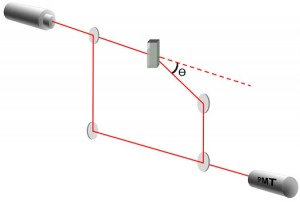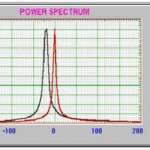• Cart
• LanguageChoose Language
• CurrencyChoose Currency

Electrophoretic light scattering is based on dynamic light scattering. Often these two techniques are combined in one instrument. In the case of DLS Brownian motions causes particles to oscillate or move in solution. With ELS an applied oscillating electric field causes particle motion. Using this method electrophoretic mobility can be measured and zeta potential can be calculated. Zeta potential is defined as the value of the electrical potential at the “shear plane” of a particle. In colloidal suspensions zeta potential will indicate if the repulsive electrical potential barrier is high enough to prevent agglomeration of particles due to short range Van der Waals forces.

The instrument setup for ELS is quite similar to DLS (Figure A). A laser beam of known wavelength passes through a cell containing conducting electrodes. Light scattered by the particles is detected by a photomultiplier (PMT). Whereas conventional dynamic light scattering techniques employs homodyne scattering principles (all of the photons of light that reach the PMT detector are scattered from the sample), ELS systems use heterodyne scattering methods in which a fraction of the laser beam is split away before reaching the sample by a mirror and directed to the PMT detector where it is combined with scattered light from particles diffusing in the sample. The fraction of redirected light is referred to as the “local oscillator” and unlike the light scattered by the sample does not fluctuate. It is used as a reference beam and must be much larger than the average intensity of the scattered light produced by the diffusing particles.Figure A

To determine zeta potential the electrophoretic mobility (µ) must first be ascertained. The mobility is a proportionality constant that relates a particle’s terminal velocity to the applied electric field (eq. 1). The frequency of the scattered light will depend on the velocity (v) of the moving particles. By superposing the reference and scattered beams a frequency shift can be obtained. From the frequency (or Doppler) shift the particle velocity can be calculated according to equation 2. Where Dn is the frequency shift, K is the same wave vector used in DLS, and v is the particle velocity. When the wave vector equation is inserted and simplified using a common trigonometric identity the equation reduces to equation 3. The mobility can then be determined using equation 4. Finally the Zeta potential x is calculated using an approximation such as the Smoluchowski formula where and hand e are the viscosity and dielectric constant of the solvent (eq. 5).

$v= \mu E (eq. 1)$

$2\pi \delta \nu = Kv (eq. 2)$

$\delta \nu = (\eta v/\lambda) sin (\theta) (eq. 3)$

$mu = (\lambda/ \eta sin \theta)(1/E)\delta\nu (eq. 4)$

$\zeta = \eta \mu\varepsilon (eq. 5)$The image shows a typical ELS power spectrum obtained for 262nnm latex spheres under and an electric field of 10V/cm. The center peak is called the reference peak with no applied electric field. The sample peak is shifted -19.3Hz which corresponds to an electrophoretic mobility of -3.61 M.U. and zeta potential of -48.8 mV assuming the Smoluchowski limit. The sample peak is broader than the reference peak indicating a range of zeta potentials within the sample rather than a single value.

In addition to the frequency power spectrum analysis, it is possible to do what is called a phase analysis light scattering (PALS) where instead of measuring frequency shift, the phase shift is determined. This technique is more sensitive than the conventional frequency measurements and can produce results with when small electric fields are applied. From the mean phase shift with respect to time (Df/Dt) electrophoretic mobility and zeta potential can be determined